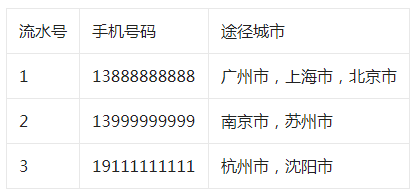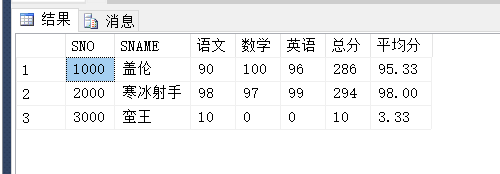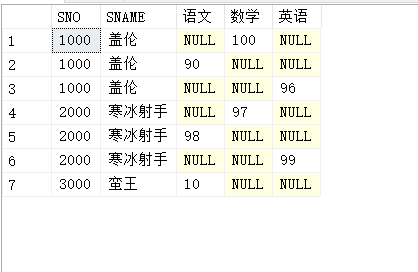# 年底了，又要搞数据报表了！整理SQL经典笔试题之行转列应用

lancelottsang

0收藏

SQL的行转列应用，主要分成俩个层面的需求，第一是数据内容的转换，第二是列级别的转换!接下来让我们一一深入地学习一下：(本案例采用的是SQLSERVER数据库)``````create function GetWayList(@uid int)
returns varchar(1000)
as
begin
declare @content varchar(1000)
set @content = ''
select @content = uwayname +','+ @content from Users_Way where uid = @uid
order by uwaytime desc
if(len(@content)>0)
begin
set @content = left(@content,len(@content)-1)
end
return @content
end``````

2.第二步直接在sql中嵌套使用即可，非常的便捷!

``SELECT uid,uphone,dbo.GetWayList(uid) as 途径城市 from Users``

``````SELECT SNO,SNAME,case subject when '语文' then score else 0 end as 语文,
case subject when '数学' then score else 0 end as 数学,
case subject when '英语' then score else 0 end as 英语
From StudentScore``````

``````SELECT SNO,SNAME,MAX(case subject when '语文' then score else 0 end) as 语文,
MAX(case subject when '数学' then score else 0 end) as 数学,
MAX(case subject when '英语' then score else 0 end) as 英语
From StudentScore
GROUP BY SNO,SNAME``````

``````SELECT SNO,SNAME,MAX(case subject when '语文' then score else 0 end) as 语文,
MAX(case subject when '数学' then score else 0 end) as 数学,
MAX(case subject when '英语' then score else 0 end) as 英语,
SUM(score) as '总分',CAST((SUM(score)*1.0/3) as decimal(18,2)) as 平均分
From StudentScore
GROUP BY SNO,SNAME``````• <聚合函数>就是我们使用的SUM,COUNT,AVG等Sql聚合函数，也就是行转列后计算列的聚合方式。
• [聚合列值]要进行聚合的列名
• [行转列前的列名]这个就是需要将行转换为列的列名。
``````SELECT SNO,SNAME,语文,数学,英语 FROM StudentScore AS T
PIVOT
(
--score为汇总成绩列
--subject 为需要转换的原始列
--(语文,数学,英语) 转换成的列名
SUM(score) for subject in(语文,数学,英语)
)TBL````````````SELECT * FROM
(
SELECT SNO,SNAME,SCORE,SUBJECT FROM StudentScore
)AS T1
PIVOT
(
SUM(score) for subject in(语文,数学,英语)
)T2
order by SNO ``````

”贫民版“行专列：

``````SELECT s.sno,s.sname,sum(ISNULL(YuWen.score,0)) as 语文,
sum(ISNULL(ShuXue.score,0)) as 数学,sum(ISNULL(YingYu.score,0)) as 英语
FROM StudentScore AS S
LEFT JOIN
(
SELECT SID,SNO,SCORE FROM StudentScore where subject = '语文'
)AS YuWen on s.sid = YuWen.sid
LEFT JOIN
(
SELECT SID,SNO,SCORE FROM StudentScore where subject = '数学'
)AS ShuXue on s.sid = ShuXue.sid
LEFT JOIN
(
SELECT SID,SNO,SCORE FROM StudentScore where subject = '英语'
)AS YingYu on s.sid = YingYu.sid
Group by s.sno,s.sname``````帖子
视频
声望
粉丝
社区精华内容